The Australian Journal of Mathematical Analysis and Applications

 Home News Editors Volumes RGMIA Subscriptions Authors Contact

ISSN 1449-5910

Sorry death is imminent for file: ../public_html/searchroot/files/tex/v19n2/v19i2p4.tex
You searched for wang
Total of 275 results found in site

52: Paper Source PDF document

Paper's Title:

An Integration Technique for Evaluating Quadratic Harmonic Sums

Author(s):

J. M. Campbell and K.-W. Chen

Department of Mathematics and Statistics,
York University, 4700 Keele St, Toronto,
ON M3J 1P3,
E-mail: jmaxwellcampbell@gmail.com

Department of Mathematics, University of Taipei,
Taipei 10048, Taiwan.
E-mail: kwchen@uTaipei.edu.tw
URL: https://math.utaipei.edu.tw/p/412-1082-22.php

Abstract:

The modified Abel lemma on summation by parts has been applied in many ways recently to determine closed-form evaluations for infinite series involving generalized harmonic numbers with an upper parameter of two. We build upon such results using an integration technique that we apply to ``convert'' a given evaluation for such a series into an evaluation for a corresponding series involving squared harmonic numbers.

32: Paper Source PDF document

Paper's Title:

Ostrowski Type Inequalities for Lebesgue Integral: a Survey of Recent Results

Author(s):

Sever S. Dragomir1,2

1Mathematics, School of Engineering & Science
Victoria University, PO Box 14428
Melbourne City, MC 8001,
Australia
E-mail: sever.dragomir@vu.edu.au

2DST-NRF Centre of Excellence in the Mathematical and Statistical Sciences,
School of Computer Science & Applied Mathematics,
University of the Witwatersrand,
Private Bag 3, Johannesburg 2050,
South Africa
URL: http://rgmia.org/dragomir

Abstract:

The main aim of this survey is to present recent results concerning Ostrowski type inequalities for the Lebesgue integral of various classes of complex and real-valued functions. The survey is intended for use by both researchers in various fields of Classical and Modern Analysis and Mathematical Inequalities and their Applications, domains which have grown exponentially in the last decade, as well as by postgraduate students and scientists applying inequalities in their specific areas.

6: Paper Source PDF document

Paper's Title:

On the Generalized Inverse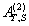over Integral Domains

Author(s):

Yaoming Yu and Guorong Wang

College of Education, Shanghai Normal University
Shanghai 200234
People's Republic of China.
yuyaoming@online.sh.cn
grwang@shnu.edu.cn

Abstract:

In this paper, we study further the generalized inverseof a matrix A over an integral domain. We give firstly some necessary and sufficient conditions for the existence of the generalized inverse, an explicit expression for the elements of the generalized inverseand an explicit expression for the generalized inverse, which reduces to the {1} inverse. Secondly, we verify that the group inverse, the Drazin inverse, the Moore-Penrose inverse and the weighted Moore-Penrose inverse are identical with the generalized inverse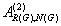for an appropriate matrix G, respectively, and then we unify the conditions for the existence and the expression for the elements of the weighted Moore-Penrose inverse, the Moore-Penrose inverse, the Drazin inverse and the group inverse over an integral domain. Thirdly, as a simple application, we give the relation between some rank equation and the existence of the generalized inverse, and a method to compute the generalized inverse. Finally, we give an example of evaluating the elements ofwithout calculating.

6: Paper Source PDF document

Paper's Title:

Positive Solutions for Systems of Three-point Nonlinear Boundary Value Problems

Author(s):

J. Henderson and S. K. Ntouyas

Department of Mathematics, Baylor University
Waco, Texas
76798-7328 USA.
Johnny_Henderson@baylor.edu
URL: http://www3.baylor.edu/~Johnny_Henderson

Department of Mathematics, University of Ioannina
451 10 Ioannina,
Greece.
sntouyas@cc.uoi.gr
URL: http://www.math.uoi.gr/~sntouyas

Abstract:

Values of λ are determined for which there exist positive solutions of the system of three-point boundary value problems, u''(t)+ λa(t)f(v(t))=0, v''(t)+λb(t)g(u(t))=0, for 0 < t <1, and satisfying, u(0) = 0, u(1)=α u(η), v(0) = 0, v(1)=α v(η). A Guo-Krasnosel'skii fixed point theorem is applied.

6: Paper Source PDF document

Paper's Title:

On a Class of Meromorphic Functions of Janowski Type Related with a Convolution Operator

Author(s):

Abdul Rahman S. Juma, Husamaldin I. Dhayea

Department of Mathematics,
Iraq.
E-mail: dr_juma@hotmail.com

Department of Mathematics,
Tikrit University, Tikrit,
Iraq.

Abstract:

In this paper, we have introduced and studied new operator \$Qkλ,m,γ by the Hadamard product (or convolution) of two linear operators Dkλ and Im,γ, then using this operator to study and investigate a new subclass of meromorphic functions of Janowski type, giving the coefficient bounds, a sufficient condition for a function to belong to the considered class and also a convolution property. The results presented provide generalizations of results given in earlier works.

6: Paper Source PDF document

Paper's Title:

Sweeping Surfaces with Darboux Frame in Euclidean 3-space E3

Author(s):

F. Mofarreh, R. Abdel-Baky and N. Alluhaibi

Mathematical Science Department, Faculty of Science,
Princess Nourah bint Abdulrahman University
Saudi Arabia.
E-mail: fyalmofarrah@pnu.edu.sa

Department of Mathematics, Faculty of Science,
University of Assiut,
Assiut 71516,
Egypt.
E-mail: rbaky@live.com

Department of Mathematics Science and Arts, College Rabigh Campus,
King Abdulaziz University
Jeddah,
Saudi Arabia.
E-mail: nallehaibi@kau.edu.sa

Abstract:

The curve on a regular surface has a moving frame and it is called Darboux frame. We introduce sweeping surfaces along the curve relating to the this frame and investigate their geometrical properties. Moreover, we obtain the necessary and sufficient conditions for these surfaces to be developable ruled surfaces. Finally, an example to illustrate the application of the results is introduced.

5: Paper Source PDF document

Paper's Title:

Positive Periodic Solutions for Second-Order Differential Equations with Generalized Neutral Operator

Author(s):

Wing-Sum Cheung, Jingli Ren and Weiwei Han

Department of Mathematics,
The University of Hong Kong
Hong Kong

Department of Mathematics, Zhengzhou University
Zhengzhou 450001,
P.R. China

wscheung@hkucc.hku.hk
renjl@zzu.edu.cn

Abstract:

By some analysis of the neutral operator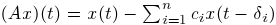and an application of the fixed-point index theorem, we obtain sufficient conditions for the existence, multiplicity and nonexistence of periodic solutions to a second-order differential equation with the prescribed neutral operator, which improve and extend some recent results of Lu-Ge, Wu-Wang, and Zhang. An example is given to illustrate our results. Moreover, the analysis of the generalized neutral operator will be helpful for other types of differential equations.

5: Paper Source PDF document

Paper's Title:

Maximal Inequalities for Multidimensionally Indexed Demimartingales and the Hßjek-Rķnyi Inequality for Associated Random Variables

Author(s):

Tasos C. Christofides and Milto Hadjikyriakou

Department of Mathematics and Statistics
University of Cyprus
P.O.Box 20537, Nicosia 1678, Cyprus

tasos@ucy.ac.cy
miltwh@gmail.com

Abstract:

Demimartingales and demisubmartingales introduced by Newman and
Wright (1982) generalize the notion of martingales and
submartingales respectively. In this paper we define
multidimensionally indexed demimartingales and demisubmartingales
and prove a maximal inequality for this general class of random
variables. As a corollary we obtain a Hßjek-Rķnyi inequality
for multidimensionally indexed associated random variables, the bound of which, when reduced to the case of single index, is sharper than the bounds already known in the literature.

5: Paper Source PDF document

Paper's Title:

New Refinements of H÷lder's Inequality

Author(s):

Xiu-Fen Ma

College of Mathematical and Computer,
No.9 of Xuefu Road, Hechuan District 401520,
Chongqing City,
The People's Republic of China.
E-mail: maxiufen86@163.com

Abstract:

In this paper, we define two mappings, investigate their properties, obtain some new refinements of H÷lder's inequality.

5: Paper Source PDF document

Paper's Title:

Iterative Algorithm for Split Generalized Mixed Equilibrium Problem Involving Relaxed Monotone Mappings in Real Hilbert Spaces

Author(s):

1U.A. Osisiogu, F.L. Adum, and 2C. Izuchukwu

1Department of Mathematics and Computer Science,
Ebonyi State University, Abakaliki,
Nigeria.

2School of Mathematics, Statistics and Computer Science,
University of KwaZulu-Natal, Durban,
South Africa.
E-mail: izuchukwuc@ukzn.ac.za, izuchukwu_c@yahoo.com

Abstract:

The main purpose of this paper is to introduce a certain class of split generalized mixed equilibrium problem involving relaxed monotone mappings. To solve our proposed problem, we introduce an iterative algorithm and obtain its strong convergence to a solution of the split generalized mixed equilibrium problems in Hilbert spaces. As special cases of the proposed problem, we studied the proximal split feasibility problem and variational inclusion problem.

5: Paper Source PDF document

Paper's Title:

Timelike Surfaces with a Common Line of Curvature in Minkowski 3-Space

Author(s):

M.K. Saad, A.Z. Ansari, M. Akram and F. Alharbi

Department of Mathematics ,
Faculty of Science,
KSA

Abstract:

In this paper, we analyze the problem of constructing a timelike surface family from a given non-null curve line of curvature. Using the Frenet frame of the non-null curve in Minkowski space E13  we express the family of surfaces as a linear combination of the components of this frame, and derive the necessary and sufficient conditions for the coefficients to satisfy both the line of curvature and the isoparametric requirements. In addition, a necessary and sufficient condition for the given non-null curve to satisfy the line of curvature and the geodesic requirements is investigated. The extension to timelike surfaces of revolution is also outlined. Meanwhile, some representative non-null curves are chosen to construct the corresponding timelike surfaces which possessing these curves as lines of curvature. Results presented in this paper have applications in geometric modeling and the manufacturing of products. In addition, some computational examples are given and plotted.

5: Paper Source PDF document

Paper's Title:

Several New Closed-form Evaluations of the Generalized Hypergeometric Function with Argument 1/16

Author(s):

B. R. Srivatsa Kumar, Insuk Kim and Arjun K. Rathie

Department of Mathematics,
Manipal Institute of Technology,
Manipal 576 104,
India.
E-mail: sri_vatsabr@yahoo.com

Department of Mathematics Education,
Wonkwang University,
Iksan, 54538,
Republic of Korea.
E-mail: iki@wku.ac.kr

Department of Mathematics,
Vedant College of Engineering and Technology,
Rajasthan Technical University,
Bundi, 323021, Rajasthan,
India.
E-mail: arjunkumarrathie@gmail.com

Abstract:

The main objective of this paper is to establish as many as thirty new closed-form evaluations of the generalized hypergeometric function q+1Fq(z) for q= 2, 3, 4. This is achieved by means of separating the generalized hypergeometric function q+1Fq(z) for q=1, 2, 3, 4, 5 into even and odd components together with the use of several known infinite series involving central binomial coefficients obtained earlier by Ji and Hei \& Ji and Zhang.

5: Paper Source PDF document

Paper's Title:

New Refinements for Integral and Sum Forms of Generalized H÷lder Inequality For N Term

Author(s):

M. Jakfar, Manuharawati, D. Savitri

Mathematics Department,
Universitas Negeri Surabaya
Jalan Ketintang Gedung C8, Surabaya, 60321
Indonesia.
manuharawati@unesa.ac.id
diansavitri@unesa.ac.id

Abstract:

We know that in the field of functional analysis, H÷lder inequality is very well known, important, and very applicable. So many researchers are interested in discussing these inequalities. Many world mathematicians try to improve these inequalities. In general, the H÷lder inequality has two forms, namely the integral form and the sum form. In this paper, we will introduce a new refinement of the generalization of H÷lder inequalities in both integral and addition forms. Especially in the sum form, improvements will be introduced that are better than the previous improvements that have been published by Jing-feng Tian, Ming-hu Ha, and Chao Wang.

4: Paper Source PDF document

Paper's Title:

Two Mappings Related to Steffensen's Inequalities

Author(s):

Liang-Cheng Wang

School of Mathematical Science,
Chongqing Institute of Technology,
Xingsheng Lu 4,
Yangjiaping 400050, Chongqing City,
China.
wangliangcheng@163.com

Abstract:

In this paper, we define two mappings closely connected with Steffensen's inequalities, investigate their main properties, give some refinements for Steffensen's inequalities and obtain new inequalities.

4: Paper Source PDF document

Paper's Title:

On a Problem on Periodic Functions

Author(s):

Mathematics Department, Faculty of Science,
Jerash Private University, Jerash,
Jordan.

Abstract:

Given a continuous periodic real function f with n translates  f1 ,..., fn , where fi(x)=f(x+ai), i=1,...,n. We solve a problem by Erdos and Chang and show that there are rational numbers r,s such that f(r)≥ fi(r), f(s)≤ fi(s), i=1,...,n. No restrictions on the constants or any further restriction on the function f are necessary as was imposed earlier.

4: Paper Source PDF document

Paper's Title:

MSplit Equality for Monotone Inclusion Problem and Fixed Point Problem in Real Banach Spaces

Author(s):

1,2Christian Chibueze Okeke, 3Abdumalik Usman Bello, 1Chinedu Izuchukwu, and 1Oluwatosin Temitope Mewomo

1School of Mathematics,
Statistics and Computer Science,
University of KwaZulu-Natal, Durban,
South Africa.
E-mail: okekec@ukzn.ac.za
E-mail: izuchukwuc@ukzn.ac.za
E-mail: mewomoo@ukzn.ac.za

2DST-NRF Center of Excellence in Mathematical and Statistical Sciences (CoE-Mass)
Johannesburg,
South Africa.

3Federal University,
Dutsin-Ma, Katsina State,
Nigeria.
E-mail: uabdulmalik@fudutsinma.edu.ng

Abstract:

In this paper a new iterative algorithm for approximating a common solution of split equality monotone inclusion problem and split equality fixed point problem is introduced. Using our algorithm, we state and prove a strong convergence theorem for approximating an element in the intersection of the set of solutions of a split equality monotone inclusion problem and the set of solutions of a split equality fixed point problem for right Bregman strongly nonexpansive mappings in the setting of p-uniformly convex Banach spaces which are also uniformly smooth. We also give some applications.

4: Paper Source PDF document

Paper's Title:

Inequalities for Functions of Selfadjoint Operators on Hilbert Spaces:
a Survey of Recent Results

Author(s):

Sever S. Dragomir1,2

1Mathematics, College of Engineering & Science
Victoria University, PO Box 14428
Melbourne City, MC 8001,
Australia
E-mail: sever.dragomir@vu.edu.au

2DST-NRF Centre of Excellence in the Mathematical and Statistical Sciences,
School of Computer Science & Applied Mathematics,
University of the Witwatersrand,
Private Bag 3, Johannesburg 2050,
South Africa
URL: https://rgmia.org/dragomir

Abstract:

The main aim of this survey is to present recent results concerning inequalities for continuous functions of selfadjoint operators on complex Hilbert spaces. It is intended for use by both researchers in various fields of Linear Operator Theory and Mathematical Inequalities, domains which have grown exponentially in the last decade, as well as by postgraduate students and scientists applying inequalities in their specific areas.

4: Paper Source PDF document

Paper's Title:

Coexisting Attractors and Bubbling Route to Chaos in Modified Coupled Duffing Oscillators

Author(s):

B. Deruni1, A. S. Hacinliyan1,2, E. Kandiran3, A. C. Keles2, S. Kaouache4, M.-S. Abdelouahab4, N.-E. Hamri4

1Department of Physics,
University of Yeditepe,
Turkey.

2Department of Information Systems and Technologies,
University of Yeditepe,
Turkey

3Department of Software Development,
University of Yeditepe,
Turkey.

4Laboratory of Mathematics and their interactions,
University Center of Abdelhafid Boussouf,
Mila 43000,
Algeria.

E-mail: berc890@gmail.com
ahacinliyan@yeditepe.edu.tr
engin.kandiran@yeditepe.edu.tr
cihan.keles@yeditepe.edu.tr
s.kaouache@centr-univ-mila.dz
medsalah3@yahoo.fr
n.hamri@centre-univ-mila.dz

Abstract:

In this article dynamical behavior of coupled Duffing oscillators is analyzed under a small modification. The oscillators have cubic damping instead of linear one. Although single duffing oscillator has complex dynamics, coupled duffing systems possess a much more complex structure. The dynamical behavior of the system is investigated both numerically and analytically. Numerical results indicate that the system has double scroll attractor with suitable parameter values. On the other hand, bifurcation diagrams illustrate rich behavior of the system, and it is seen that, system enters into chaos with different routes. Beside classical bifurcations, bubbling route to chaos is observed for suitable parameter settings. On the other hand, Multistability of the system is indicated with the coexisting attractors, such that under same parameter setting the system shows different periodic and chaotic attractors. Moreover, chaotic synchronization of coupled oscillators is illustrated in final section.

4: Paper Source PDF document

Paper's Title:

Fractional Integral Inequalities of Hermite-Hadamard Type for P-convex and Quasi-Convex Stochastic Process

Author(s):

Oualid Rholam, Mohammed Barmaki and Driss Gretet

National School of Applied Sciences (ENSA),
University Ibn Tofail,
B.P 242 Kenitra 14000,
phone number : +212606257757,
Morocco.
E-mail: oualid.rholam@uit.ac.ma

Science Faculty Ben M'sik,
University Hassan II,
B.P 7955 Av Driss El Harti Sidi Othmane 20700,
phone number : +212 5 22 70 46 71 ,
Morocco.
E-mail:  mohammed.barmaki@uit.ac.ma

National School of Applied Sciences (ENSA),
University Ibn Tofail,
B.P 242 Kenitra 14000,
phone number : +212661403557,
Morocco.
E-mail: driss.gretete@uit.ac.ma

Abstract:

In this paper we consider the class of P-convex and Quasi-convex stochastic processes on witch we apply a general class of generalized fractional integral operator in order to establish new integral inequalities of Hermite-Hadammard type. then we obtain some results for well known types of fractional integrals. Results obtained in this paper may be starting point as well as a useful source of inspiration for further research in convex analysis.

3: Paper Source PDF document

Paper's Title:

Positive Solutions of Evolution Operator Equations

Author(s):

Department of Applied Mathematics,
Babes-Bolyai University,
Cluj, Romania

Abstract:

Existence and localization results are derived from KrasnoselskiiÆs compressionexpansion fixed point theorem in cones, for operator equations in spaces of continuous functions from a compact real interval to an abstract space. The main idea, first used in , is to handle two equivalent operator forms of the equation, one of fixed point type giving the operator to which KrasnoselskiiÆs theorem applies and an other one of coincidence type which is used to localize a positive solution in a shell. An application is presented for a boundary value problem associated to a fourth order partial differential equation on a rectangular domain.

3: Paper Source PDF document

Paper's Title:

A Generalization of Ostrowski's Inequality for Functions of Bounded Variation via a Parameter

Author(s):

Seth Kermausuor

Department of Mathematics and Computer Science,
Alabama State University,
Montgomery, AL 36101,
USA.
E-mail: skermausour@alasu.edu

Abstract:

In this paper, we provide a generalization of the Ostrowski's inequality for functions of bounded variation for k points via a parameter λ∈[0,1]. As a by product, we consider some particular cases to obtained some interesting inequalities in these directions. Our results generalizes some of the results by Dragomir in [S. S. DRAGOMIR, The Ostrowski inequality for mappings of bounded variation, Bull. Austral. Math. Soc., 60 (1999), pp. 495--508.]

3: Paper Source PDF document

Paper's Title:

Fractional exp(-φ(ξ))- Expansion Method and its Application to Space--Time Nonlinear Fractional Equations

Author(s):

A. A. Moussa and L. A. Alhakim

Department of Management Information System and Production Management,
College of Business and Economics, Qassim University,
P.O. BOX 6666, Buraidah: 51452,
Saudi Arabia.
E-mail: Alaamath81@gmail.com

Department of Management Information System and Production Management,
College of Business and Economics, Qassim University,
P.O. BOX 6666, Buraidah: 51452,
Saudi Arabia.
E-mail: Lama2736@gmail.com

Abstract:

In this paper, we mainly suggest a new method that depends on the fractional derivative proposed by Katugampola for solving nonlinear fractional partial differential equations. Using this method, we obtained numerous useful and surprising solutions for the space--time fractional nonlinear Whitham--Broer--Kaup equations and space--time fractional generalized nonlinear Hirota--Satsuma coupled KdV equations. The solutions obtained varied between hyperbolic, trigonometric, and rational functions, and we hope those interested in the real-life applications of the previous two equations will find this approach useful.

3: Paper Source PDF document

Paper's Title:

Reduced Generalized Combination Synchronization Between Two n-Dimensional Integer-Order Hyperchaotic Systems and One m-Dimensional Fractional-Order Chaotic System

Author(s):

Smail Kaouache, Mohammed Salah Abdelouahab and Rabah Bououden

Laboratory of Mathematics and their interactions,
Abdelhafid Boussouf University Center, Mila.
Algeria
E-mail: smailkaouache@gmail.com, medsalah3@yahoo.fr, rabouden@yahoo.fr

Abstract:

This paper is devoted to investigate the problem of reduced generalized combination synchronization (RGCS) between two n-dimensional integer-order hyperchaotic drive systems and one m-dimensional fractional-order chaotic response system. According to the stability theorem of fractional-order linear system, an active mode controller is proposed to accomplish this end. Moreover, the proposed synchronization scheme is applied to synchronize three different chaotic systems, which are the Danca hyperchaotic system, the modified hyperchaotic Rossler system, and the fractional-order Rabinovich-Fabrikant chaotic system. Finally, numerical results are presented to fit our theoretical analysis.

3: Paper Source PDF document

Paper's Title:

Existence and Approximation of Traveling Wavefronts for the Diffusive Mackey-Glass Equation

Author(s):

C. Ramirez-Carrasco and J. Molina-Garay

Chile
E-mail: carloshrc1989@gmail.com
molina@imca.edu.pe

Abstract:

In this paper, we consider the diffusive Mackey-Glass model with discrete delay. This equation describes the dynamics of the blood cell production. We investigate the existence of traveling wavefronts solutions connecting the two steady states of the model. We develop an alternative proof of the existence of such solutions and we also demonstrate the existence of traveling wavefronts moving at minimum speed. The proposed approach is based on the use technique of upper-lower solutions. Finally, through an iterative procedure, we show numerical simulations that approximate the traveling wavefronts, thus confirming our theoretical results.

3: Paper Source PDF document

Paper's Title:

Evaluation of a New Class of Double Integrals Involving Generalized Hypergeometric Function 4F3

Author(s):

Joohyung Kim, Insuk Kim and Harsh V. Harsh

Department of Mathematics Education,
Wonkwang University, Iksan, 570-749,
Korea.
E-mail: joohyung@wku.ac.kr

Department of Mathematics Education,
Wonkwang University, Iksan, 570-749,
Korea.
E-mail: iki@wku.ac.kr

Department of Mathematics, Amity School of Eng. and Tech.,
Amity University Rajasthan
NH-11C, Jaipur-303002, Rajasthan,
India.
E-mail: harshvardhanharsh@gmail.com

Abstract:

Very recently, Kim evaluated some double integrals involving a generalized hypergeometric function 3F2 with the help of generalization of Edwards's well-known double integral due to Kim,  et al. and generalized classical Watson's summation theorem obtained earlier by Lavoie,  et al. In this research paper we evaluate one hundred double integrals involving generalized hypergeometric function 4F3 in the form of four master formulas (25 each) viz. in the most general form for any integer. Some interesting results have also be obtained as special cases of our main findings.

3: Paper Source PDF document

Paper's Title:

A Review on Minimally Supported Frequency Wavelets

Author(s):

K Pallavi1, M C Lineesh1, A Noufal2

1Department of Mathematics,
National Institute of Technology Calicut,
Kerala 673601,
India.
E-mail:
pavikrishnakumar@gmail.com
lineesh@nitc.ac.in

2Department of Mathematics,
Cochin University of Science and Technology,
Kerala 682022,
India.
E-mail: noufal@cusat.ac.in

Abstract:

This paper provides a review on Minimally Supported Frequency (MSF) wavelets that includes the construction and characterization of MSF wavelets. The characterization of MSF wavelets induced from an MRA is discussed and the nature of the low-pass filter associated with it is explained. The concept of wavelet set and dimension function is introduced to study this class of wavelets. Along with MSF wavelets, s-elementary wavelets and unimodular wavelets are also considered due to the similarity in definitions. Examples and illustrations are provided for more clarity.

3: Paper Source PDF document

Paper's Title:

Estimation for Bounded Solutions of Some Nonlinear Integral Inequalities with Delay in Several Variables

Author(s):

Smakdji Mohamed Elhadi, Denche Mouhamed and Khellaf Hassane

Department of Mathematics
University of FrĶres Mentouri
PO Box 25000, Ain Elbay,
Constantine,
Algeria.
E-mail: khellafhassane@umc.edu.dz

Abstract:

In this paper, some new nonlinear retarded integral inequalities of Gronwall-Bellman type for functions of two and n-independents variables are investigated. The derived results can be applied in the study of differential-integral equations with time delay. An example is given to illustrate the application of our results.

3: Paper Source PDF document

Paper's Title:

SQIRV Model for Omicron Variant with Time Delay

Author(s):

S. Dickson, S. Padmasekaran, G. E. Chatzarakis and S. L. Panetsos

Mathematics, Periyar University, Periyar Palkalai Nagar, Salem,
India.

Electrical and Electronic Engineering Educators, School of
Pedagogical and Technological Education (ASPETE),
Marousi 15122, Athens,
Greece.
E-mail: geaxatz@otenet.gr, spanetsos@aspete.gr

Abstract:

In order to examine the dynamics of the Omicron variant, this paper uses mathematical modelling and analysis of a SQIRV model, taking into account the delay in the conversion of susceptible individuals into infected individuals and infected individuals into recovered individuals. The pandemic was eventually controlled as a result of the massive delays. To assure the safety of the host population, this concept incorporates quarantine and the COVID-19 vaccine. Both local and global stability of the model are examined. It is found that the fundamental reproduction number affects both local and global stability conditions. Our findings show that asymptomatic cases caused by an affected population play an important role in increasing Omicron infection in the general population. The most recent data on the pandemic Omicron variant from Tamil Nadu, India, is verified.

2: Paper Source PDF document

Paper's Title:

On an Extension of HilbertÆs Integral Inequality with Some Parameters

Author(s):

Bicheng Yang

Department of Mathematics, Guangdong Education College, Guangzhou, Guangdong 510303, PeopleÆs Republic of China.
URL
:

Abstract:

In this paper, by introducing some parameters and estimating the weight function, we give an extension of HilbertÆs integral inequality with a best constant factor. As applications, we consider the equivalent form and some particular results.

2: Paper Source PDF document

Paper's Title:

Norm Estimates for the Difference between BochnerÆs Integral and the Convex Combination of FunctionÆs Values

Author(s):

P. Cerone, Y.J. Cho, S.S. Dragomir, J.K. Kim, and S.S. Kim

School of Computer Science and Mathematics,
Victoria University of Technology,
Po Box 14428, Mcmc 8001, Victoria, Australia.

URL
:

Department of Mathematics Education, College of Education,
Gyeongsang National University, Chinju 660-701, Korea

School of Computer Science and Mathematics,
Victoria University of Technology,
Po Box 14428, Mcmc 8001, Victoria, Australia.

URL
:

Department of Mathematics, Kyungnam University,
Masan,, Kyungnam 631-701, Korea

Department of Mathematics, Dongeui University,
Pusan 614-714, Korea

Abstract:

Norm estimates are developed between the Bochner integral of a vector-valued function in Banach spaces having the Radon-Nikodym property and the convex combination of function values taken on a division of the interval [a, b].

2: Paper Source PDF document

Paper's Title:

A New Hardy-Hilbert's Type Inequality for Double Series and its Applications

Author(s):

Mingzhe Gao

Department of Mathematics and Computer Science, Normal College Jishou University,
Jishou Hunan, 416000,
People's Republic of China
mingzhegao1940@yahoo.com.cn

Abstract:

In this paper, it is shown that a new Hardy-HilbertÆs type inequality for double series can be established by introducing a parameter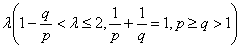and the weight function of the form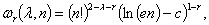where c is Euler constant and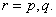And the coefficient is proved to be the best possible. And as the mathematics aesthetics, several important constants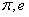and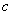appear simultaneously in the coefficient and the weight function when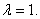In particular, for case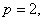some new HilbertÆs type inequalities are obtained. As applications, some extensions of Hardy-LittlewoodÆs inequality are given.

2: Paper Source PDF document

Paper's Title:

Oscillations of First Order Linear Delay Difference Equations

Author(s):

G. E. Chatzarakis and I. P. Stavroulakis

Department of Mathematics, University of Ioannina,
451 10, Greece
ipstav@cc.uoi.gr

Abstract:

Consider the first order linear delay difference equation of the form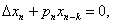Ā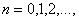Āwhere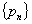Āis a sequence of nonnegative real numbers, k is a positive integer and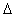Ādenotes the forward difference operator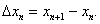ĀNew oscillation criteria are established when the well-known oscillation conditions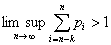Āand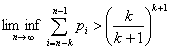Āare not satisfied. The results obtained essentially improve known results in the literature.

2: Paper Source PDF document

Paper's Title:

A Stability of the G-type Functional Equation

Author(s):

Gwang Hui Kim

Department of Mathematics, Kangnam University
Suwon 449-702, Korea.
ghkim@kangnam.ac.kr

Abstract:

We will investigate the stability in the sense of Găvruţă for the G-type functional equation f(φ(x))=Γ(x)f(x)+ψ(x) and the stability in the sense of Ger for the functional equation of the form f(φ(x))=Γ(x)f(x). As a consequence, we obtain a stability results for G-function equation.

2: Paper Source PDF document

Paper's Title:

The Iterated Variational Method for the Eigenelements of a Class of Two-Point Boundary Value Problems

Author(s):

Muhammed I. Syam and Qasem M. Al-Mdallal

Department of Mathematical Sciences, College of Science, UAE University,
P. O. Box 17551, Al-Ain,
United Arab Emirates
Q.Almdallal@uaeu.ac.ae
M.Syam@uaeu.ac.ae

Abstract:

The iterated variational method is considered in the approximation of eigenvalues and eigenfunctions for a class of two point boundary value problems. The implementation of this method is easy and competes well with other methods. Numerical examples are presented to show the efficiency of the method proposed. Comparison with the work of others is also illustrated.

2: Paper Source PDF document

Paper's Title:

Existence of Solutions for Third Order Nonlinear Boundary Value Problems

Author(s):

Yue Hu and Zuodong Yang

School of Mathematics and Computer Science, Nanjing Normal University, Jiangsu Nanjing 210097,
China.
huu3y2@163.com

College of Zhongbei, Nanjing Normal University, Jiangsu Nanjing 210046,
China.
zdyang_jin@263.net
yangzuodong@njnu.edu.cn

Abstract:

In this paper, the existence of solution for a class of third order quasilinear ordinary differential equations with nonlinear boundary value problems

p(u"))'=f(t,u,u',u"), u(0)=A, u'(0)=B, R(u'(1),u"(1))=0

is established. The results are obtained by using upper and lower solution methods.

2: Paper Source PDF document

Paper's Title:

Nontrivial Solutions of Singular Superlinear Three-point Boundary Value Problems at Resonance

Author(s):

Feng Wang, Fang Zhang

School of Mathematics and Physics,
Changzou University,
Changzhou, 213164,
China.
fengwang188@163.com

Abstract:

The singular superlinear second order three-point boundary value problems at resonance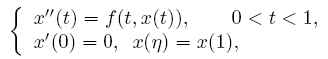are considered under some conditions concerning the first eigenvalues corresponding to the relevant linear operators, where n ∈ (0,1) is a constant, f is allowed to be singular at both t=0 and t=1. The existence results of nontrivial solutions are given by means of the topological degree theory.

2: Paper Source PDF document

Paper's Title:

Two Remarks on Commutators of Hardy Operator

Author(s):

Yasuo Komori-Furuya

School of High Technology for Human Welfare
Tokai University
317 Nishino Numazu, Shizuoka 410-0395 Japan
komori@wing.ncc.u-tokai.ac.jp

Abstract:

Fu and Lu showed that
the commutator of multiplication operator by b and
the n-dimensional Hardy operator
is bounded on Lp if b is in some CMO space.
We shall prove the converse of this theorem
and also prove that their result is optimal by giving a counterexample

2: Paper Source PDF document

Paper's Title:

On a Hilbert-type Inequality with the Polygamma Function

Author(s):

Bing He and Bicheng Yang
Department of Mathematics, Guangdong Education College,
Guangzhou, 510303,
China.

Department of Mathematics, Guangdong Education College,
Guangzhou, 510303,
China.

Abstract:

By applying the method of weight function and the technique of real analysis, a Hilbert-type inequality with a best constant factor is established, where the best constant factor is made of the polygamma function. Furthermore, the inverse form is given.

2: Paper Source PDF document

Paper's Title:

Hermite-Hadamard-Fejer Type Inequalities for Harmonically s-convex Functions via Fractional Integrals

Author(s):

İmdat İşcan, Mehmet Kunt

Department of Mathematics,
Faculty of Sciences and Arts,
Giresun University, Giresun,
Turkey.
E-mail: imdat.iscan@giresun.edu.tr

Department of Mathematics,
Faculty of Sciences,
61080, Trabzon,
Turkey.
E-mail: mkunt@ktu.edu.tr

Abstract:

In this paper, some Hermite-Hadamard-Fejer type integral inequalities for harmonically s-convex functions in fractional integral forms have been obtained.

2: Paper Source PDF document

Paper's Title:

Presentation a mathematical model for bone metastases control by using tamoxifen

Author(s):

Maryam Nikbakht, Alireza Fakharzadeh Jahromi and Aghileh Heydari

Department of Mathematics,
Payame Noor University,
P.O.Box 19395-3697, Tehran,
Iran.
.E-mail: maryam_nikbakht@pnu.ac.ir

Department of Mathematics,
Faculty of Basic Science,
Shiraz University of Technology.

Department of Mathematics,
Payame Noor University,
P.O.Box 19395-3697, Tehran,
Iran.
E-mail: a-heidari@pnu.ac.ir

Abstract:

Bone is a common site for metastases (secondary tumor) because of breast and prostate cancer. According to our evaluations the mathematical aspect of the effect of drug in bone metastases has not been studied yet. Hence, this paper suggested a new mathematical model for bone metastases control by using tamoxifen. The proposed model is a system of nonlinear partial differential equations. In this paper our purpose is to present a control model for bone metastases. At end by some numerical simulations, the proposed model is examined by using physician.

2: Paper Source PDF document

Paper's Title:

Existence of Positive Solutions for Nonlinear Fractional Differential Equations with Multi-point Boundary Conditions

Author(s):

Khenchela University, Department of Mathematics,
Khenchela, 40000,
Algeria.

Abstract:

This paper is devoted to the existence results of positive solutions for a nonlinear fractional differential equations with multi-point boundary conditions. By means of the Schauder fixed point theorem, some results on the existence are obtained.

2: Paper Source PDF document

Paper's Title:

The Functional Equation With an Exponential Polynomial Solution and its Stability

Author(s):

Young Whan Lee1, Gwang Hui Kim2*, and Jeong Il Kim3

1Department of Computer Hacking and Information Security,
College of Engineering, Daejeon University,
Daejeon, 34520,
Korea(Republic of).
E-mail: ywlee@dju.ac.kr

2Department of Mathematics,
Kangnam University,
Yongin, Gyeonggi, 16979,
Korea(Republic of).
E-mail: i
ghkim@kangnam.ac.kr

3Department of Statistics,
Daejeon University,
Daejeon 34520,
Korea(Republic of).
E-mail: jlkim@dju.ac.kr

Abstract:

In this paper, we prove that the unique continuous solution of the functional equationiswhere pn(x) is a polynomial with degree n andWe also obtain the superstability and stability of the functional equation with the following forms, respectively:and2: Paper Source PDF document

Paper's Title:

Some Inequalities of the Hermite-Hadamard Type for k-Fractional Conformable Integrals

Author(s):

C.-J. Huang, G. Rahman, K. S. Nisar, A. Ghaffar and F. Qi

Department of Mathematics, Ganzhou Teachers College,
Ganzhou 341000, Jiangxi,
China.
E-mail: hcj73jx@126.com , huangcj1973@qq.com

Department of Mathematics, Shaheed Benazir Bhutto University,
Sheringal, Upper Dir, Khyber Pakhtoonkhwa,
Pakistan.
E-mail: gauhar55uom@gmail.com

Department of Mathematics, College of Arts and Science at Wadi Aldawaser, 11991,
Prince Sattam Bin Abdulaziz University, Riyadh Region,
Kingdom of Saudi Arabia.
E-mail: n.sooppy@psau.edu.sa, ksnisar1@gmail.com

Department of Mathematical Science, Balochistan University of Information Technology,
Engineering and Management Sciences, Quetta,
Pakistan.
E-mail: abdulghaffar.jaffar@gmail.com

School of Mathematical Sciences, Tianjin Polytechnic University,
Tianjin 300387,
China; Institute of Mathematics,
Henan Polytechnic University, Jiaozuo 454010, Henan,
China.
E-mail: qifeng618@gmail.com, qifeng618@qq.com

Abstract:

In the paper, the authors deal with generalized k-fractional conformable integrals, establish some inequalities of the Hermite-Hadamard type for generalized k-fractional conformable integrals for convex functions, and generalize known inequalities of the Hermite-Hadamard type for conformable fractional integrals.

2: Paper Source PDF document

Paper's Title:

Cubic Alternating Harmonic Number Sums

Author(s):

Anthony Sofo

Victoria University,
College of Engineering and Science,
Melbourne City,
Australia.
E-mail: Anthony.Sofo@vu.edu.au

Abstract:

We develop new closed form representations of sums of cubic alternating harmonic numbers and reciprocal binomial coefficients. We also identify a new integral representation for the ζ (4)  constant.

2: Paper Source PDF document

Paper's Title:

Dynamical Analysis of HIV/AIDS Epidemic Model with Two Latent Stages, Vertical Transmission and Treatment

Author(s):

Nur Shofianah, Isnani Darti, Syaiful Anam

Mathematics Department,Faculty of Mathematics and Natural Sciences.
University of Brawijaya,
Jl. Veteran, Malang 65145,
Indonesia.
E-mail: nur_shofianah@ub.ac.id, isnanidarti@ub.ac.id, syaiful@ub.ac.id

Abstract:

We discuss about dynamical analysis of HIV/AIDS epidemic model with two latent stages, vertical transmission and treatment. In this model, the spreading of HIV occurs through both horizontal and vertical transmission. There is also treatment for individual who has been HIV infected. The latent stage is divided into slow and fast latent stage based on the immune condition which varies for each individual. Dynamical analysis result shows that the model has two equilibrium points: the disease-free equilibrium point and the endemic equilibrium point. The existence and global stability of equilibrium points depend on the basic reproduction number R0. When R0 <1, only the disease-free equilibrium point exists. If R0 >1, there are two equilibrium points, which are the disease-free equilibrium point and the endemic equilibrium point. Based on the result of stability analysis, the disease-free equilibrium point is globally asymptotically stable if R0 <1, while if R0 > 1 and p=q, the endemic equilibrium point will be globally asymptotically stable. In the end, we show some numerical simulations to support the analytical result.

2: Paper Source PDF document

Paper's Title:

On an extension of Edwards's double integral with applications

Author(s):

I. Kim, S. Jun, Y. Vyas and A. K. Rathie

Department of Mathematics Education,
Wonkwang University,
Iksan, 570-749,
Republic of Korea.

General Education Institute,
Konkuk University,
Chungju 380-701,
Republic of Korea.

Department of Mathematics, School of Engineering,
Bhatewar, Udaipur, 313601, Rajasthan State,
India.

Department of Mathematics,
Vedant College of Engineering and Technology,
(Rajasthan Technical University),
Bundi-323021, Rajasthan,
India.

E-mail: iki@wku.ac.kr sjun@kku.ac.kr
yashoverdhan.vyas@spsu.ac.in arjunkumarrathie@gmail.com

Abstract:

The aim of this note is to provide an extension of the well known and useful Edwards's double integral. As an application, new class of twelve double integrals involving hypergeometric function have been evaluated in terms of gamma function. The results are established with the help of classical summation theorems for the series 3F2 due to Watson, Dixon and Whipple. Several new and interesting integrals have also been obtained from our main findings.

2: Paper Source PDF document

Paper's Title:

On Ruled Surfaces According to Quasi-Frame in Euclidean 3-Space

Author(s):

M. Khalifa Saad and R. A. Abdel-Baky

Department of Mathematics, Faculty of Science,
KSA.
Department of Mathematics, Faculty of Science,
Sohag University, Sohag,
EGYPT.
E-mail: mohamed_khalifa77@science.sohag.edu.eg, mohammed.khalifa@iu.edu.sa

Department of Mathematics, Faculty of Science,
Assiut University, Assiut,
EGYPT.
E-mail: rbaky@live.com

Abstract:

This paper aims to study the skew ruled surfaces by using the quasi-frame of Smarandache curves in the Euclidean 3-space. Also, we reveal the relationship between Serret-Frenet and quasi-frames and give a parametric representation of a directional ruled surface using the quasi-frame. Besides, some comparative examples are given and plotted which support our method and main results.

2: Paper Source PDF document

Paper's Title:

Optimal Control Analysis of HIV/AIDS Epidemic Model with an Antiretroviral Treatment

Author(s):

U. Habibah and R. A. Sari

Mathematics Department and Reseach Group of Biomathematics,
Faculty of Mathematics and Natural Science,
Brawijaya University, Jl. Veteran Malang 65145,
Indonesia.
E-mail: ummu_habibah@ub.ac.id

Abstract:

A mathematical model of HIV/AIDS is governed by a system of ordinary differential equations in the presence of an antiretroviral treatment (ARV). The theory of optimal control is applied to an epidemic model of HIV/AIDS which an ARV is used as a control strategy in order to prevent the spread of HIV/AIDS. The optimality system is derived by applying the Pontryagin's Minimum Principle. We analyze the boundedness and positivity of solutions, and an existence of the optimal control. Numerical simulations are conducted to obtain numerical solution of the optimally system.

2: Paper Source PDF document

Paper's Title:

A New Method with Regularization for Solving Split Variational Inequality Problems in Real Hilbert Spaces

Author(s):

Francis Akutsah1 and Ojen Kumar Narain2

1School of Mathematics,
Statistics and Computer Science,
University of KwaZulu-Natal, Durban,
South Africa.
E-mail: 216040405@stu.ukzn.ac.za, akutsah@gmail.com

2School of Mathematics,
Statistics and Computer Science,
University of KwaZulu-Natal, Durban,
South Africa.
E-mail: naraino@ukzn.ac.za

Abstract:

In this paper, we introduce a new inertial extrapolation method with regularization for approximating solutions of split variational inequality problems in the frame work of real Hilbert spaces. We prove that the proposed method converges strongly to a minimum-norm solution of the problem without using the conventional two cases approach. In addition, we present some numerical experiments to show the efficiency and applicability of the proposed method. The results obtained in this paper extend, generalize and improve several results in this direction.

2: Paper Source PDF document

Paper's Title:

Additive Mappings on Semiprime Rings Functioning as Centralizers

Author(s):

Abu Zaid Ansari and Faiza Shujat

Department of Mathematics,
Faculty of Science,
K.S.A.
E-mail: ansari.abuzaid@gmail.com, ansari.abuzaid@iu.edu.sa

Department of Mathematics,
Faculty of Science,
K.S.A.
E-mail: faiza.shujat@gmail.com, fullahkhan@taibahu.edu.sa

Abstract:

The objective of this research is to prove that an additive mapping T:R R is a centralizer on R if it satisfies any one of the following identities: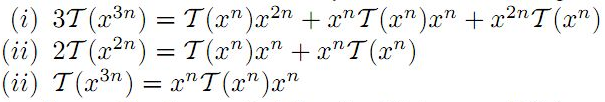for all x R, where n 1 is a fixed integer and R is any suitably torsion free semiprime ring. Some results on involution "*" are also presented as consequences of the main theorems. In addition, we will take criticism in account with examples.

2: Paper Source PDF document

Paper's Title:

Higher Order Accurate Compact Schemes for Time Dependent Linear and Nonlinear Convection-Diffusion Equations

Author(s):

S. Thomas, Gopika P.B. and S. K. Nadupuri

Department of Mathematics
National Institute of Technology Calicut
Kerala
673601
India.
E-mail: sobinputhiyaveettil@gmail.com pbgopika@gmail.com nsk@nitc.ac.in

Abstract:

The primary objective of this work is to study higher order compact finite difference schemes for finding the numerical solution of convection-diffusion equations which are widely used in engineering applications. The first part of this work is concerned with a higher order exponential scheme for solving unsteady one dimensional linear convection-diffusion equation. The scheme is set up with a fourth order compact exponential discretization for space and cubic \$C^1\$-spline collocation method for time. The scheme achieves fourth order accuracy in both temporal and spatial variables and is proved to be unconditionally stable. The second part explores the utility of a sixth order compact finite difference scheme in space and Huta's improved sixth order Runge-Kutta scheme in time combined to find the numerical solution of one dimensional nonlinear convection-diffusion equations. Numerical experiments are carried out with Burgers' equation to demonstrate the accuracy of the new scheme which is sixth order in both space and time. Also a sixth order in space predictor-corrector method is proposed. A comparative study is performed of the proposed schemes with existing predictor-corrector method. The investigation of computational order of convergence is presented.

1: Paper Source PDF document

Paper's Title:

A general theory of decision making

Author(s):

Frank Hansen

Department of Economics,
University of Copenhagen,
Studiestraede 6, DK-1455 Copenhagen K
Denmark Frank.Hansen@econ.ku.dk
URL: http://www.econ.ku.dk/okofh

Abstract:

We formulate a general theory of decision making based on a lattice of observable events, and we exhibit a large class of representations called the general model. Some of the representations are equivalent to the so called standard model in which observable events are modelled by an algebra of measurable subsets of a state space, while others are not compatible with such a description. We show that the general model collapses to the standard model, if and only if an additional axiom is satisfied. We argue that this axiom is not very natural and thus assert that the standard model may not be general enough to model all relevant phenomena in economics. Using the general model we are (as opposed to Schmeidler ) able to rationalize Ellsberg's paradox without the introduction of non-additive measures.

1: Paper Source PDF document

Paper's Title:

Positive Periodic Time-Scale Solutions for Functional Dynamic Equations

Author(s):

Douglas R. Anderson and Joan Hoffacker

Department of Mathematics and Computer Science
Concordia College
andersod@cord.edu
URL:
http://www.cord.edu/faculty/andersod/

Department of Mathematical Sciences
Clemson University
Clemson, SC 29634 USA
johoff@clemson.edu
URL:
http://www.math.clemson.edu/facstaff/johoff.htm

Abstract:

Using Krasnoselskii's fixed point theorem, we establish the existence of positive periodic solutions to two pairs of related nonautonomous functional delta dynamic equations on periodic time scales, and then extend the discussion to higher-dimensional equations. Two pairs of corresponding nabla equations are also provided in an analogous manner.

1: Paper Source PDF document

Paper's Title:

On Vector Variational Inequality Problem in Terms of Bifunctions

Author(s):

C. S. Lalitha and Monika Mehta

Department of Mathematics, Rajdhani College,
University of Delhi, Raja Garden,
Delhi 110015, India
cslalitha@rediffmail.com

Department of Mathematics, Satyawati College,
University Of Delhi, Ashok Vihar,
Phase-III, Delhi 110052, India
mridul_in@yahoo.com

Abstract:

In this paper, we consider a generalized vector variational inequality problem expressed in terms of a bifunction and establish existence theorems for this problem by using the concepts of cone convexity and cone strong quasiconvexity and employing the celebrated Fan's Lemma. We also give two types of gap functions for this problem.

1: Paper Source PDF document

Paper's Title:

A Strengthened Hardy-Hilbert's Type Inequality

Author(s):

Weihong Wang and Bicheng Yang

Department of Mathematics, Guangdong Education Institute,
Guangzhou, Guangdong 520303,
People's Republic Of China
wwh@gdei.edu.cn
bcyang@pub.guangzhou.gd.cn
URL: http://www1.gdei.edu.cn/yangbicheng/index.html

Abstract:

By using the improved Euler-Maclaurin's summation formula and estimating the weight coefficient, we give a new strengthened version of the more accurate Hardy-Hilbert's type inequality. As applications, a strengthened version of the equivalent form is considered.

1: Paper Source PDF document

Paper's Title:

The Superstability of the Pexider Type Trigonometric Functional Equation

Author(s):

Gwang Hui Kim and Young Whan Lee

Department of Mathematics, Kangnam
University Yongin, Gyeonggi, 446-702, Korea.
ghkim@kangnam.ac.kr

Department of Computer and Information Security
Daejeon University, Daejeon 300-716, Korea.
ywlee@dju.ac.kr

Abstract:

The aim of this paper is to investigate the stability problem for
the Pexider type (hyperbolic) trigonometric functional equation
f(x+y)+f(x+σy)=λg(x)h(y) under the conditions :
|f(x+y)+f(x+σy)- λg(x)h(y)|≤φ(x),
φ(y)
, and min {φ(x), φ (y)}.

As a consequence, we have generalized the results of stability for
the cosine(d'Alembert), sine, and the Wilson functional equations by J.
Baker, P. Găvruta, R. Badora and R. Ger, Pl.~Kannappan, and G.
H. Kim

1: Paper Source PDF document

Paper's Title:

Approximation of an AQCQ-Functional Equation and its Applications

Author(s):

Choonkil Park and Jung Rye Lee

Department of Mathematics,
Research Institute for Natural Sciences,
Hanyang University, Seoul 133-791,
Korea;

Department of Mathematics,
Daejin University,
Kyeonggi 487-711,
Korea

baak@hanyang.ac.kr
jrlee@daejin.ac.kr

Abstract:

This paper is a survey on the generalized Hyers-Ulam stability of an AQCQ-functional equation in several spaces. Its content is divided into the following sections:

1. Introduction and preliminaries.

2. Generalized Hyers-Ulam stability of an AQCQ-functional equation in Banach spaces: direct method.

3. Generalized Hyers-Ulam stability of an AQCQ-functional equation in Banach spaces: fixed point method.

4. Generalized Hyers-Ulam stability of an AQCQ-functional equation in random Banach spaces: direct method.

5. Generalized Hyers-Ulam stability of an AQCQ-functional equation in random Banach spaces: fixed point method.

6. Generalized Hyers-Ulam stability of an AQCQ-functional equation in non-Archi-medean Banach spaces: direct method.

7. Generalized Hyers-Ulam stability of an AQCQ-functional equation in non-Archi-medean Banach spaces: fixed point method.

1: Paper Source PDF document

Paper's Title:

Finite and Infinite Order Solutions of a Class of Higher Order Linear Differential Equations

Author(s):

Department of Mathematics,
Laboratory of Pure and Applied Mathematics,
University of Mostaganem, B. P 227 Mostaganem,
ALGERIA

Abstract:

In this paper, we investigate the growth of solutions of higher order linear differential equations where most of the coefficients have the same order and type with each other.

1: Paper Source PDF document

Paper's Title:

Schwarz Method for Variational Inequalities Related to Ergodic Control Problems

Author(s):

Department of Mathematics, Badji Mokhtar University, Annaba 23000,
P.O.Box. 12, Annaba 23000, Algeria

signor_2000@yahoo.fr

Mcherihalima@yahoo.fr

Abstract:

In this paper, we study variational inequalities related to ergodic control problems studied by M. BoulbrachĶne and H. Sissaoui , where the "discount factor" (i.e., the zero order term) is set to 0, we use an overlapping Schwarz method on nomatching grid which consists in decomposing the domain in two subdomains. For α ∈ ]0.1[ we provide the discretization on each subdomain converges in L -norm.

1: Paper Source PDF document

Paper's Title:

L∞- Error Estimate of Schwarz Algorithm for Elliptic Quasi-Variational Inequalities Related to Impulse Control Problem

Author(s):

Lab. LANOS, Department of Mathematics,
P.O.Box 12, Annaba 23000,
Algeria.

Lab. LAIG, Department of Mathematics,
University May 8th 1945,
P.O.Box 401, Guelma 24000,
Algeria.

allmehri@yahoo.fr

Abstract:

In this work, we study Schwarz method for a class of elliptic quasi-variational inequalities. The principal result of this investigation is to prove the error estimate in ∞-norm for two domains with overlapping nonmatching grids, using the geometrical convergence, and the uniform convergence of Cortey Dumont.

1: Paper Source PDF document

Paper's Title:

Some Gr³ss Type Inequalities in Inner Product Spaces

Author(s):

Sever S. Dragomir1,2

1Mathematics, School of Engineering & Science
Victoria University, PO Box 14428
Melbourne City, MC 8001,
Australia
E-mail: sever.dragomir@vu.edu.au

2School of Computer Science & Applied Mathematics,
University of the Witwatersrand,
Private Bag 3, Johannesburg 2050,
South Africa

URL: http://rgmia.org/dragomir

Abstract:

Some inequalities in inner product spaces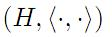that provide upper bounds for the quantities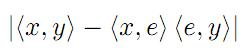and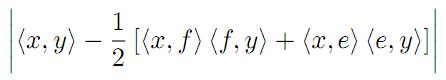,

where e,f ∈ H with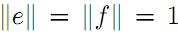and x,y are vectors in H satisfying some appropriate assumptions are given. Applications for discrete and integral inequalities are provided as well.

1: Paper Source PDF document

Paper's Title:

Hermite-Hadamard Type Inequalities for k-Riemann Liouville Fractional Integrals Via Two Kinds of Convexity

Author(s):

R. Hussain1, A. Ali2, G. Gulshan3, A. Latif4 and K. Rauf5

1,2,3,4Department of Mathematics,
Mirpur University of Science and Technology, Mirpur.
Pakistan.
E-mail1rashida12@gmail.com
E-mail2: unigraz2009@yahoo.com
E-mail3: ghazalagulshan@yahoo.com
E-mail4: asialatif87@gmail.com

5Department of Mathematics,
University of Ilorin, Ilorin,
Nigeria.
E-mail5: krauf@unilorin.edu.ng

Abstract:

In this article, a fundamental integral identity including the first order derivative of a given function via k-Riemann-Liouville fractional integral is established. This is used to obtain further Hermite-Hadamard type inequalities involving left-sided and right-sided k-Riemann-Liouville fractional integrals for m-convex and (s,m)-convex functions respectively.

1: Paper Source PDF document

Paper's Title:

Mapped Chebyshev Spectral Methods for Solving Second Kind Integral Equations on the Real Line

Author(s):

Ahmed Guechi and Azedine Rahmoune

Department of Mathematics, University of Bordj Bou Arrķridj,
El Anasser, 34030, BBA,
Algeria.
E-mail: a.guechi2017@gmail.com
E-mail: a.rahmoune@univ-bba.dz

Abstract:

In this paper we investigate the utility of mappings to solve numerically an important class of integral equations on the real line. The main idea is to map the infinite interval to a finite one and use Chebyshev spectral-collocation method to solve the mapped integral equation in the finite interval. Numerical examples are presented to illustrate the accuracy of the method.

1: Paper Source PDF document

Paper's Title:

Inequalities for Discrete F-Divergence Measures: A Survey of Recent Results

Author(s):

Sever S. Dragomir1,2

1Mathematics, School of Engineering & Science
Victoria University, PO Box 14428
Melbourne City, MC 8001,
Australia
E-mail: sever.dragomir@vu.edu.au

2DST-NRF Centre of Excellence in the Mathematical and Statistical Sciences,
School of Computer Science & Applied Mathematics,
University of the Witwatersrand,
Private Bag 3, Johannesburg 2050,
South Africa
URL: http://rgmia.org/dragomir

Abstract:

In this paper we survey some recent results obtained by the author in providing various bounds for the celebrated f-divergence measure for various classes of functions f. Several techniques including inequalities of Jensen and Slater types for convex functions are employed. Bounds in terms of Kullback-Leibler Distance, Hellinger Discrimination and Varation distance are provided. Approximations of the f-divergence measure by the use of the celebrated Ostrowski and Trapezoid inequalities are obtained. More accurate approximation formulae that make use of Taylor's expansion with integral remainder are also surveyed. A comprehensive list of recent papers by several authors related this important concept in information theory is also included as an appendix to the main text.

1: Paper Source PDF document

Paper's Title:

A Comparison Between Two Different Stochastic Epidemic Models with Respect to the Entropy

Author(s):

Department of Mathematics,
University of Sussex,
Brighton BN1 9QH,
UK.
E-mail: f.fatehi@sussex.ac.uk
URL: http://www.sussex.ac.uk/profiles/361251

Department of Pure Mathematics, Faculty of Mathematics and Computer,
Shahid Bahonar University of Kerman,
Kerman 76169-14111,
Iran.

Abstract:

In this paper at first a brief history of mathematical models is presented with the aim to clarify the reliability of stochastic models over deterministic models. Next, the necessary background about random variables and stochastic processes, especially Markov chains and the entropy are introduced. After that, entropy of SIR stochastic models is computed and it is proven that an epidemic will disappear after a long time. Entropy of a stochastic mathematical model determines the average uncertainty about the outcome of that random experiment. At the end, we introduce a chain binomial epidemic model and compute its entropy, which is then compared with the DTMC SIR epidemic model to show which one is nearer to reality.

1: Paper Source PDF document

Paper's Title:

Polynomial Dichotomy of C0-Quasi Semigroups in Banach Spaces

Author(s):

Sutrima1,2, Christiana Rini Indrati2, Lina Aryati2

1Department of Mathematics,
Universitas Sebelas Maret,
PO Box 57126, Surakarta,
Indonesia.
E-mail: sutrima@mipa.uns.ac.id

2Department of Mathematics,
PO Box 55281, Yogyakarta,
Indonesia.
E-mail: rinii@ugm.ac.id, lina@ugm.ac.id

Abstract:

Stability of solutions of the problems is an important aspect for application purposes. Since its introduction by Datko , the concept of exponential stability has been developed in various types of stability by various approaches. The existing conditions use evolution operator, evolution semigroup, and quasi semigroup approach for the non-autonomous problems and a semigroup approach for the autonomous cases. However, the polynomial stability based on C0-quasi semigroups has not been discussed in the references. In this paper we propose a new stability for C0-quasi semigroups on Banach spaces i.e the polynomial stability and polynomial dichotomy. As the results, the sufficient and necessary conditions for the polynomial and uniform polynomial stability are established as well as the sufficiency for the polynomial dichotomy. The results are also confirmed by the examples.

1: Paper Source PDF document

Paper's Title:

A Multivalued Version of the Radon-Nikodym Theorem, via the Single-valued Gould Integral

Author(s):

Domenico Candeloro1, Anca Croitoru2, Alina Gavriluţ2, Anna Rita Sambucini1

1Dept. of Mathematics and Computer Sciences,
University of Perugia,
1, Via Vanvitelli -- 06123, Perugia,
Italy.
E-mail:  domenico.candeloro@unipg.it, anna.sambucini@unipg.it

2Faculty of Mathematics,
Al. I. Cuza University,
700506 Iaşi,
Romania.
E-mail: croitoru@uaic.ro, gavrilut@uaic.ro

Abstract:

In this paper we consider a Gould type integral of real functions with respect to a compact and convex valued not necessarily additive measure. In particular we will introduce the concept of integrable multimeasure and, thanks to this notion, we will establish an exact Radon-Nikodym theorem relative to a fuzzy multisubmeasure which is new also in the finite dimensional case. Some results concerning the Gould integral are also obtained.

1: Paper Source PDF document

Paper's Title:

The Conservativeness of Girsanov Transformed for Symmetric Jump-diffusion Process

Author(s):

Mila Kurniawaty and Marjono

Department of Mathematics,
Universitas Brawijaya,
Malang,
Indonesia.
E-mail: mila_n12@ub.ac.id, marjono@ub.ac.id

Abstract:

We study about the Girsanov transformed for symmetric Markov processes with jumps associated with regular Dirichlet form. We prove the conservativeness of it by dividing the regular Dirichlet form  into the "small jump" part and the "big jump" part.

1: Paper Source PDF document

Paper's Title:

On a New Class of Eulerian's Type Integrals Involving Generalized Hypergeometric Functions

Author(s):

Sungtae Jun, Insuk Kim and Arjun K. Rathie

General Education Institute,
Konkuk University, Chungju 380-701,
Republic of Korea.

Department of Mathematics Education,
Wonkwang University, Iksan, 570-749,
Republic of Korea.

Department of Mathematics,
Vedant College of Engineering and Technology (Rajasthan Technical University),
Bundi-323021, Rajasthan,
India.

E-mail: sjun@kku.ac.kr, iki@wku.ac.kr, arjunkumarrathie@gmail.com

Abstract:

Very recently Masjed-Jamei and Koepf established interesting and useful generalizations of various classical summation theorems for the 2F1, 3F2, 4F3, 5F4 and 6F5 generalized hypergeometric series. The main aim of this paper is to establish eleven Eulerian's type integrals involving generalized hypergeometric functions by employing these theorems. Several special cases have also been given.

1: Paper Source PDF document

Paper's Title:

Some Convergence Results forĀ Jungck-Am Iterative Process In Hyperbolic Spaces

Author(s):

Akindele Adebayo Mebawondu and Oluwatosin Temitope Mewomo

School of Mathematics, Statistics and Computer Science,
University of KwaZulu-Natal, Durban,
South Africa.
E-mail: 216028272@stu.ukzn.ac.za, mewomoo@ukzn.ac.za

Abstract:

In this paper, we introduce a new three steps iterative process called Jungck-AM iterative process and show that the proposed iterative process can be used to approximate fixed points of Jungck-contractive type mappings and Jungck-Suzuki type mappings. In addition, we establish some strong and Δ-convergence results for the approximation of fixed points of Jungck-Suzuki type mappings in the frame work of uniformly convex hyperbolic space. Furthermore, we show that the newly proposed iterative process has a better rate of convergence compare to the Jungck-Noor, Jungck-SP, Jungck-CR and some existing iterative processes in the literature. Finally, stability, data dependency results for Jungck-AM iterative process is established and we present an analytical proof and numerical examples to validate our claim.

1: Paper Source PDF document

Paper's Title:

A Low Order Least-Squares Nonconforming Finite Element Method for Steady Magnetohydrodynamic Equations

Author(s):

Z. Yu, D. Shi and H. Zhu

College of Science,
Zhongyuan University of Technology,
Zhengzhou 450007,
China.
E-mail: 5772@zut.edu.cn

School of Mathematics and Statistics,
Zhengzhou University,
Zhengzhou 450001,
China.
E-mail: shi_dy@126.com

Mathematics Department,
University of Southern Mississippi,
Hattiesburg MS, 39406,
U.S.A
E-mail: huiqing.zhu@usm.edu

Abstract:

A low order least-squares nonconforming finite element (NFE) method is proposed for magnetohydrodynamic equations with EQ1rot element and zero-order Raviart-Thomas element. Based on the above element's typical interpolations properties, the existence and uniqueness of the approximate solutions are proved and the optimal order error estimates for the corresponding variables are derived.

1: Paper Source PDF document

Paper's Title:

Some New Mappings Related to Weighted Mean Inequalities

Author(s):

Xiu-Fen Ma

College of Mathematical and Computer,
No.9 of Xuefu Road, Hechuan District 401520,
Chongqing City,
The People's Republic of China.
E-mail: maxiufen86@163.com

Abstract:

In this paper, we define four mappings related to weighted mean inequalities, investigate their properties, and obtain some new refinements of weighted mean inequalities.

1: Paper Source PDF document

Paper's Title:

Generalised Models for Torsional Spine Reconnection

Author(s):

Ali Khalaf Hussain Al-Hachami

Department of Mathematics,
College of Education For Pure Sciences,
Wasit University,
Iraq.
E-mail: alhachamia@uowasit.edu.iq

Abstract:

Three-dimensional (3D) null points are available in wealth in the solar corona, and the equivalent is probably going to be valid in other astrophysical situations. On-going outcomes from sun oriented perceptions and from reproductions propose that reconnection at such 3D nulls may assume a significant job in the coronal dynamics. The properties of the torsional spine method of magnetic reconnection at 3D nulls are researched. Kinematic model are created, which incorporate the term ηJ that is spatially localised around the null, stretching out along the spine of the null. The null point is to research the impact of shifting the level of asymmetry of the null point magnetic field on the subsequent reconnection process where past examinations constantly considered a non-nonexclusive radially symmetric null. Specifically we analyse the rate of reconnection of magnetic flux at the spine of null point. Logical arrangements are determined for the enduring kinematic equation, and contrasted and the after effects of torsional spine reconnection models when the current is restricted in which the Maxwell conditions are illuminated. The geometry of the current layers inside which torsional spine reconnection happen is autonomous on the symmetry of the magnetic field. Torsional spine reconnection happens in a thin cylinder around the spine, with circular cross-segment when the fan eigenvalues are extraordinary. The short axis of the circle being along the solid field bearing. Just as it was discovered that the fundamental structure of the method of attractive reconnection considered is unaffected by changing the magnetic field symmetry, that is, the plasma flow is discovered rotational around the spine of null point. The spatiotemporal pinnacle current, and the pinnacle reconnection rate achieved, are found not to rely upon the level of asymmetry.

1: Paper Source PDF document

Paper's Title:

Analysis of a Frictional Contact Problem for Viscoelastic Piezoelectric Materials

Author(s):

Meziane Said Ameur, Tedjani Hadj Ammar and Laid Maiza

Departement of Mathematics,
El Oued University,
P.O. Box 789, 39000 El Oued,
Algeria.
E-mail: said-ameur-meziane@univ-eloued.dz

Departement of Mathematics,
El Oued University,
P.O. Box 789, 39000 El Oued,
Algeria.

Department of Mathematics,
Kasdi Merbah University,
30000 Ouargla,
Algeria.
E-mail: maiza.laid@univ-ouargla.dz

Abstract:

In this paper, we consider a mathematical model that describes the quasi-static process of contact between two thermo-electro-viscoelastic bodies with damage and adhesion. The damage of the materials caused by elastic deformations. The contact is frictional and modeled with a normal compliance condition involving adhesion effect of contact surfaces. Evolution of the bonding field is described by a first order differential equation. We derive variational formulation for the model and prove an existence and uniqueness result of the weak solution. The proof is based on arguments of evolutionary variational inequalities, parabolic inequalities, differential equations, and fixed point theorem.

1: Paper Source PDF document

Paper's Title:

Double Difference of Composition Operator on Bloch Spaces

Author(s):

Rinchen Tundup

Department of Mathematics
University of Jammu
Jammu and Kashmir
India.

E-mail: joneytun123@gmail.com

Abstract:

In this paper we characterize the compactness of double difference of three non-compact composition operators on Bloch space induced by three holomorphic self maps on the unit disc.

1: Paper Source PDF document

Paper's Title:

Numerical Approximation by the Method of Lines with Finite-volume Approach of a Solute Transport Equation in Periodic Heterogeneous Porous Medium

Author(s):

D. J. Bambi Pemba and B. Ondami

Universitķ Marien Ngouabi,
Factutķ des Sciences et Techniques,
BP 69, Brazzaville,
Congo.
E-mail: bondami@gmail.com

Abstract:

In this paper we are interested in the numerical approximation of a two-dimensional solute transport equation in heterogeneous porous media having periodic structures. It is a class of problems which has been the subject of various works in the literature, where different methods are proposed for the determination of the so-called homogenized problem. We are interested in this paper, in the direct resolution of the problem, and we use the method of lines with a finite volume approach to discretize this equation. This discretization leads to an ordinary differential equation (ODE) that we discretize by the Euler implicit scheme. Numerical experiments comparing the obtained solution and the homogenized problem solution are presented. They show that the precision and robustness of this method depend on the ratio between, the mesh size and the parameter involved in the periodic homogenization.

1: Paper Source PDF document

Paper's Title:

Solving Non-Autonomous Nonlinear Systems of Ordinary Differential Equations Using Multi-Stage Differential Transform Method

Author(s):

K. A. Ahmad, Z. Zainuddin, F. A. Abdullah

School of Mathematical Sciences Universiti Sains Malaysia
11800 USM Penang
Malaysia.
zarita@usm.my
farahaini@usm.my

Abstract:

Differential equations are basic tools to describe a wide variety of phenomena in nature such as, electrostatics, physics, chemistry, economics, etc. In this paper, a technique is developed to solve nonlinear and linear systems of ordinary differential equations based on the standard Differential Transform Method (DTM) and Multi-stage Differential Transform Method (MsDTM). Comparative numerical results that we are obtained by MsDTM and Runge-Kutta method are proposed. The numerical results showed that the MsDTM gives more accurate approximation as compared to the Runge-Kutta numerical method for the solutions of nonlinear and linear systems of ordinary differential equations

1: Paper Source PDF document

Paper's Title:

Generalized Von Neumann-Jordan Constant for Morrey Spaces and Small Morrey Spaces

Author(s):

H. Rahman and H. Gunawan

Department of Mathematics,
Islamic State University Maulana Malik Ibrahim Malang,
Jalan Gajayana No.50,
Indonesia.
E-mail: hairur@mat.uin-malang.ac.id

Analysis and Geometry Group,
Faculty of Mathematics and Natural Sciences,
Bandung Institute of Technology, Bandung 40132,
Indonesia.
E-mail: hgunawan@math.itb.ac.id
URL:  http://personal.fmipa.itb.ac.id/hgunawan/

Abstract:

In this paper we calculate some geometric constants for Morrey spaces and small Morrey spaces, namely generalized Von Neumann-Jordan constant, modified Von Neumann-Jordan constants, and Zbaganu constant. All these constants measure the uniformly nonsquareness of the spaces. We obtain that their values are the same as the value of Von Neumann-Jordan constant for Morrey spaces and small Morrey spaces.

1: Paper Source PDF document

Paper's Title:

Nonlinear System of Mixed Ordered Variational Inclusions Involving XOR Operation

Author(s):

Iqbal Ahmad, Abdullah and Syed Shakaib Irfan

Department of Mechanical Engineering,
College of Engineering, Qassim University
Buraidah 51452, Al-Qassim,
Saudi Arabia.

Zakir Husain Delhi College,
University of Delhi,
JLN Marg, New Delhi- 110 002,
India.
E-mail: abdullahdu@qec.edu.sa

Department of Mathematics,
Aligarh Muslim University, Aligarh,
India.
E-mail: shakaibirfan@gmail.com

Abstract:

In this work, we introduce and solve an NSMOVI frameworks system involving XOR operation with the help of a proposed iterative algorithm in real ordered positive Hilbert spaces. We discuss the existence of a solution of a considered system of inclusions involving XOR operation by applying the resolvent operator technique with XOR operation and also study the strong convergence of the sequences generated by the considered algorithm. Further, we give a numerical example in support of our considered problem which gives the grantee that all the proposed conditions of our main result are fulfilled.

1: Paper Source PDF document

Paper's Title:

On Euler's First Transformation Formula for k-hypergeometric Function

Author(s):

Sungtae Jun and Insuk Kim

General Education Institute,
Konkuk University, Chungju 380-701,
Republic of Korea.
E-mail: sjun@kku.ac.kr

Department of Mathematics Education,
Wonkwang University, Iksan, 570-749,
Republic of Korea.
E-mail: iki@wku.ac.kr

Abstract:

Mubeen et al. obtained Kummer's first transformation for the k-hypergeometric function. The aim of this note is to provide the Euler-type first transformation for the k-hypergeometric function. As a limiting case, we recover the results of Mubeen et al. In addition to this, an alternate and easy derivation of Kummer's first transformation for the k-hypergeometric function is also given.

1: Paper Source PDF document

Paper's Title:

Coefficient Estimates Of Sakaguchi Kind Functions Using Lucas Polynomials

Author(s):

H. Priya and B. Srutha Keerthi

Department of Mathematics,
VIT Chennai Campus,
Chennai - 600 048,
India.
E-mail: priyaharikrishnan18@gmail.com

Department of Mathematics,
VIT Chennai Campus,
Chennai - 600 048,
India.
E-mail: i
sruthilaya06@yahoo.co.in

Abstract:

By means of (p,q) Lucas polynomials, we estimate coefficient bounds and Fekete-Szego inequalities for functions belonging to this class. Several corollaries and consequences of the main results are also obtained.

1: Paper Source PDF document

Paper's Title:

Error Bounds for Numerical Integration of Functions of Lower Smoothness and Gauss-Legendre Quadrature Rule

Author(s):

Samuel A. Surulere and Abiola O. Oladeji

Tshwane University of Technology
Department of Mathematics and Statistics
175, Nelson Mandela drive, Arcadia, Pretoria,
South Africa.
E-mail: samuel.abayomi.sas@gmail.com

Abstract:

The error bounds of the rectangular, trapezoidal and Simpson's rules which are commonly used in approximating the integral of a function (f(x)) over an interval ([a,b]) were estimated. The error bounds of the second, and third generating functions of the Gauss-Legendre quadrature rules were also estimated in this paper. It was shown that for an (f(t)) whose smoothness is increasing, the accuracy of the fourth, sixth and eighth error bound of the second, and third generating functions of the Gauss-Legendre quadrature rule does not increase. It was also shown that the accuracy of the fourth error bound of the Simpson's (1/3) and (3/8) rules does not increase.

1: Paper Source PDF document

Paper's Title:

Conservativeness Criteria of Girsanov Transformation for Non-Symmetric Jump-diffusion

Author(s):

Mila Kurniawaty

DDepartment of Mathematics,
Universitas Brawijaya, Malang,
Indonesia.
E-mail: mila_n12@ub.ac.id

Abstract:

We develop the condition in our previous paper [The Conservativeness of Girsanov transformed for symmetric jump-diffusion process (2018)] in the framework of nonsymmetric Markov process with jumps associated with regular Dirichlet form. We prove the conservativeness of it by relation in duality of Girsanov transformed process and recurrent criteria of Dirichlet form.

1: Paper Source PDF document

Paper's Title:

A Caratheodory's Approximate Solutions of Stochastic Differential Equations Under the H÷lder Condition

Author(s):

Bo-Kyeong Kim and Young-Ho Kim

Department of Mathematics,
Changwon National University,
Changwon, Gyeongsangnam-do 51140,
Korea.
E-mail: claire9576@naver.com
yhkim@changwon.ac.kr

Abstract:

In this paper, based on the theorem of the uniqueness of the solution of the stochastic differential equation, the convergence possibility of the Caratheodory's approximate solution was studied by approximating the unique solution. To obtain this convergence theorem, we used a H÷lder condition and a weakened linear growth condition. Furthermore, The auxiliary theorems for the existence and continuity of the Caratheodory's approximate solution were investigated as a prerequisite.

1: Paper Source PDF document

Paper's Title:

On the Class of Totally Polynomially Posinormal Operators

Author(s):

E. Shine Lal, T. Prasad, P. Ramya

Department of Mathematics,University College,
Thiruvananthapuram, Kerala, 695034.
India.
E-mail: shinelal.e@gmail.com

Department of Mathematics,
University of Calicut,
Malapuram, Kerala 673635,
India.

Department of Mathematics,
N.S.S College,
Nemmara, Kerala, 678508
India.
E-mail: ramyagcc@gmail.com

Abstract:

In this paper, we proved that if  T B(H)  is totally P-posinormal operator with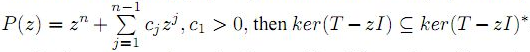. Moreover, we study spectral continuity and range kernel orthogonality of these class of operators.

1: Paper Source PDF document

Paper's Title:

Linear System of Singularly Perturbed Initial Value Problems with Robin Initial Conditions

Author(s):

S. Dinesh, G. E. Chatzarakis, S. L. Panetsos and S. Sivamani

Department of Mathematics,
Saranathan College of Engineering,
Tiruchirappalli-620012,
India.

Department of Electrical and Electronic Engineering Educators,
School of Pedagogical and Technological Education,
Marousi 15122, Athens,
Greece.

E-mail: geaxatz@otenet.gr, dineshselvaraj24@gmail.com,
spanetsos@aspete.gr, winmayi2012@gmail.com

Abstract:

On the interval (0,1], this paper considers an initial value problem for a system of n singularly perturbed differential equations with Robin initial conditions. On a piecewise uniform Shishkin mesh, a computational approach based on a classical finite difference scheme is proposed. This approach is shown to be first-order convergent in the maximum norm uniformly in the perturbation parameters. The theory is illustrated by a numerical example.

Search and serve lasted 0 second(s).

® 2004-2023 Austral Internet Publishing